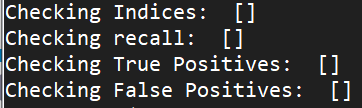# RetinaNet Out of Bounds Error

Hello folks, I’m having some trouble with my model. When I train it I get the error below.

If anyone know how to resolve this that would be great and thanks in advance.

I’ve been trying to follow this implementation of RetinaNet with a Resnet-101 backbone:

`
mAP:
myClass: 0.0

pytorch-retinanet/train.py", line 218, in
main()
pytorch-retinanet/train.py", line 205, in main
mAP = csv_eval.evaluate(dataset_val, retinanet)

pytorch-retinanet/retinanet/csv_eval.py", line 240, in evaluate
print("Precision: ",precision[-1])
IndexError: index -1 is out of bounds for axis 0 with size 0
`

My losses with a lr=1e-9 started off like:

``````Epoch: 0 | Iteration: 0 | Classification loss: 0.05783 | Regression loss: 0.00000 | Running loss: 0.05783
Epoch: 0 | Iteration: 1 | Classification loss: 0.05783 | Regression loss: 0.00000 | Running loss: 0.05783
Epoch: 0 | Iteration: 2 | Classification loss: 0.05783 | Regression loss: 0.00000 | Running loss: 0.05783
Epoch: 0 | Iteration: 3 | Classification loss: 0.05783 | Regression loss: 0.00000 | Running loss: 0.05783
Epoch: 0 | Iteration: 4 | Classification loss: 0.05783 | Regression loss: 0.00000 | Running loss: 0.05783
Epoch: 0 | Iteration: 5 | Classification loss: 0.05783 | Regression loss: 0.00000 | Running loss: 0.05783
Epoch: 0 | Iteration: 6 | Classification loss: 0.05783 | Regression loss: 0.00000 | Running loss: 0.05783
Epoch: 0 | Iteration: 7 | Classification loss: 0.05783 | Regression loss: 0.00000 | Running loss: 0.05783
Epoch: 0 | Iteration: 8 | Classification loss: 0.05783 | Regression loss: 0.00000 | Running loss: 0.05783
Epoch: 0 | Iteration: 9 | Classification loss: 0.05783 | Regression loss: 0.00000 | Running loss: 0.05783
Epoch: 0 | Iteration: 10 | Classification loss: 0.05783 | Regression loss: 0.00000 | Running loss: 0.05783
Epoch: 0 | Iteration: 11 | Classification loss: 0.05782 | Regression loss: 0.00000 | Running loss: 0.05783
Epoch: 0 | Iteration: 12 | Classification loss: 0.05782 | Regression loss: 0.00000 | Running loss: 0.05783
Epoch: 0 | Iteration: 13 | Classification loss: 0.05782 | Regression loss: 0.00000 | Running loss: 0.05783
Epoch: 0 | Iteration: 14 | Classification loss: 0.05782 | Regression loss: 0.00000 | Running loss: 0.05783
Epoch: 0 | Iteration: 15 | Classification loss: 0.05782 | Regression loss: 0.00000 | Running loss: 0.05783
Epoch: 0 | Iteration: 16 | Classification loss: 0.05782 | Regression loss: 0.00000 | Running loss: 0.05783
Epoch: 0 | Iteration: 17 | Classification loss: 0.05782 | Regression loss: 0.00000 | Running loss: 0.05783
Epoch: 0 | Iteration: 18 | Classification loss: 0.05782 | Regression loss: 0.00000 | Running loss: 0.05783
Epoch: 0 | Iteration: 19 | Classification loss: 0.05782 | Regression loss: 0.00000 | Running loss: 0.05783

``````

`precision` is computed as:

``````precision = true_positives / np.maximum(true_positives + false_positives, np.finfo(np.float64).eps)
``````

while `true_positives` is defined as an array containing ones and zeros depending on the `detections`:

``````            for d in detections:
scores = np.append(scores, d)

if annotations.shape == 0:
false_positives = np.append(false_positives, 1)
true_positives  = np.append(true_positives, 0)
continue

overlaps            = compute_overlap(np.expand_dims(d, axis=0), annotations)
assigned_annotation = np.argmax(overlaps, axis=1)
max_overlap         = overlaps[0, assigned_annotation]

if max_overlap >= iou_threshold and assigned_annotation not in detected_annotations:
false_positives = np.append(false_positives, 0)
true_positives  = np.append(true_positives, 1)
detected_annotations.append(assigned_annotation)
else:
false_positives = np.append(false_positives, 1)
true_positives  = np.append(true_positives, 0)
``````

Based on this it seems `detections` might be empty or just a single value, so check why this would be the case and add a few conditions if needed.

Thank you very much @ptrblck for trying to help, I’ve been stuck on these problems for weeks and weeks and every time I think I’m getting a little closer … something happens.

I’ve managed to upload my files to GitHub and wondered if you saw anything obvious that might be causing the problem, I’d love to learn how to solve the issue.

I just wanted to train a custom RetinaNet model with a Resnet-101 backbone on my own data which has only 1 class.

So I tried to do some print statements like you kindly suggested:I’ve logged everything here:

I printed out the detections, annotations, generator, retinanet, score threshold (0.05), max detections (100).

even changing the score threshold to 0.01 gives me the same output.

I’ve even printed the data as a tensor which is in that mylog.txt file

I even used the anchor optomisation tool to get the best anchors just in case that was the issue and using the recommended anchors did nothing to improve the result, the identical problem still reamins.